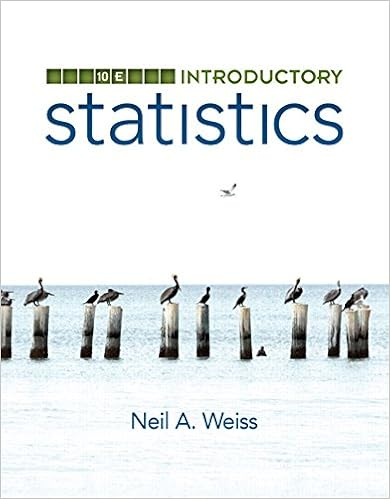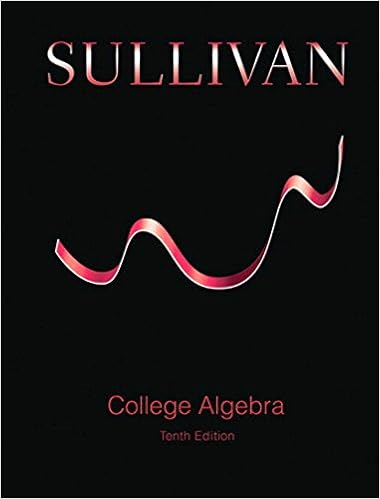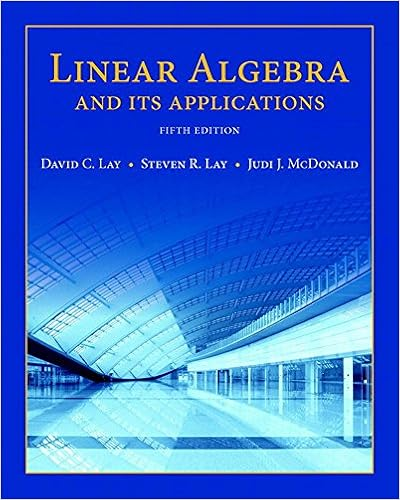# Homework 7 - Homework 7 - Math 142, Frank Thorne...

• Homework Help
• PresidentHackerIbex3077
• 1

This preview shows page 1 out of 1 page.

The preview shows page 1 - 1 out of 1 page.
Homework 7 - Math 142, Frank Thorne ([email protected])Due Friday, October 11(a) What is a parametric curve? Draw a picture and give an example.(b) Given a curve defined by parametric equationsx=f(t),y=g(t), which variables are functionsof the other variables?(c) Give (and draw) examples of parametric curves that illustrate the following possibilities. Ex-plain thoroughly.ycan be described as a function ofx.xcan be described as a function ofy.Both of the above are true.Neither of the above are true.(d) Suppose you have a functiony=f(x) that you want to define by parametric equations. Howwould you do it?Could you do it a different way?Is there any limit to the number of ways you could do it?(e) Give parametric equations for a line, a circle, an ellipse (other than a circle), and a parabola.Graph all of your curves.(f) A bug sits on the edge of a bicycle tire while you ride the bicycle, and does not move (relativeto its position on the tire). Assume that the bicycle moves forward at a constant speed and
##### We have textbook solutions for you!The document you are viewing contains questions related to this textbook.
Chapter 10 / Exercise 1
Calculus
StewartExpert VerifiedBrowse all Textbook Solutions
End of preview. Want to read the entire page?

Course Hero member to access this document

Term
Winter
Professor
NoProfessor
Tags
Parametric equation, Conic section, Frank Thorne, thornef mailbox sc edu
##### We have textbook solutions for you!
The document you are viewing contains questions related to this textbook.The document you are viewing contains questions related to this textbook.
Chapter 10 / Exercise 1
Calculus
StewartExpert Verified
•••